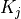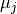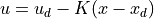# control.create_statefbk_iosystem¶

control.create_statefbk_iosystem(sys, gain, integral_action=None, estimator=None, controller_type=None, xd_labels=None, ud_labels=None, gainsched_indices=None, gainsched_method='linear', control_indices=None, state_indices=None, name=None, inputs=None, outputs=None, states=None, **kwargs)[source]

Create an I/O system using a (full) state feedback controller

This function creates an input/output system that implements a state feedback controller of the form

u = ud - K_p (x - xd) - K_i integral(C x - C x_d)

It can be called in the form

ctrl, clsys = ct.create_statefbk_iosystem(sys, K)

where sys is the process dynamics and K is the state (+ integral) feedback gain (eg, from LQR). The function returns the controller ctrl and the closed loop systems clsys, both as I/O systems.

A gain scheduled controller can also be created, by passing a list of gains and a corresponding list of values of a set of scheduling variables. In this case, the controller has the form

u = ud - K_p(mu) (x - xd) - K_i(mu) integral(C x - C x_d)

where mu represents the scheduling variable.

Parameters
• sys (InputOutputSystem) – The I/O system that represents the process dynamics. If no estimator is given, the output of this system should represent the full state.

• gain (ndarray or tuple) –

If an array is given, it represents the state feedback gain (K). This matrix defines the gains to be applied to the system. If integral_action is None, then the dimensions of this array should be (sys.ninputs, sys.nstates). If integral action is set to a matrix or a function, then additional columns represent the gains of the integral states of the controller.

If a tuple is given, then it specifies a gain schedule. The tuple should be of the form (gains, points) where gains is a list of gainsand points is a list of valuesat which the gains are computed. The gainsched_indices parameter should be used to specify the scheduling variables.

• xd_labels (str or list of str, optional) – Set the name of the signals to use for the desired state and inputs. If a single string is specified, it should be a format string using the variable i as an index. Otherwise, a list of strings matching the size of xd and ud, respectively, should be used. Default is “xd[{i}]” for xd_labels and “ud[{i}]” for ud_labels. These settings can also be overriden using the inputs keyword.

• ud_labels (str or list of str, optional) – Set the name of the signals to use for the desired state and inputs. If a single string is specified, it should be a format string using the variable i as an index. Otherwise, a list of strings matching the size of xd and ud, respectively, should be used. Default is “xd[{i}]” for xd_labels and “ud[{i}]” for ud_labels. These settings can also be overriden using the inputs keyword.

• integral_action (ndarray, optional) – If this keyword is specified, the controller can include integral action in addition to state feedback. The value of the integral_action keyword should be an ndarray that will be multiplied by the current and desired state to generate the error for the internal integrator states of the control law.

• estimator (InputOutputSystem, optional) – If an estimator is provided, use the states of the estimator as the system inputs for the controller.

• gainsched_indices (int, slice, or list of int or str, optional) – If a gain scheduled controller is specified, specify the indices of the controller input to use for scheduling the gain. The input to the controller is the desired state xd, the desired input ud, and the system state x (or state estimate xhat, if an estimator is given). If value is an integer q, the first q values of the [xd, ud, x] vector are used. Otherwise, the value should be a slice or a list of indices. The list of indices can be specified as either integer offsets or as signal names. The default is to use the desired state xd.

• gainsched_method (str, optional) – The method to use for gain scheduling. Possible values are ‘linear’ (default), ‘nearest’, and ‘cubic’. More information is available in scipy.interpolate.griddata(). For points outside of the convex hull of the scheduling points, the gain at the nearest point is used.

• controller_type ('linear' or 'nonlinear', optional) – Set the type of controller to create. The default for a linear gain is a linear controller implementing the LQR regulator. If the type is ‘nonlinear’, a :class:NonlinearIOSystem is created instead, with the gain K as a parameter (allowing modifications of the gain at runtime). If the gain parameter is a tuple, then a nonlinear, gain-scheduled controller is created.

• control_indices (int, slice, or list of int or str, optional) – Specify the indices of the system inputs that should be determined by the state feedback controller. If value is an integer m, the first m system inputs are used. Otherwise, the value should be a slice or a list of indices. The list of indices can be specified as either integer offsets or as system input signal names. If not specified, defaults to the system inputs.

• state_indices (int, slice, or list of int or str, optional) – Specify the indices of the system (or estimator) outputs that should be used by the state feedback controller. If value is an integer n, the first n system states are used. Otherwise, the value should be a slice or a list of indices. The list of indices can be specified as either integer offsets or as estimator/system output signal names. If not specified, defaults to the system states.

• inputs (str, or list of str, optional) – List of strings that name the individual signals of the transformed system. If not given, the inputs and outputs are the same as the original system.

• outputs (str, or list of str, optional) – List of strings that name the individual signals of the transformed system. If not given, the inputs and outputs are the same as the original system.

• name (string, optional) – System name. If unspecified, a generic name <sys[id]> is generated with a unique integer id.

Returns

• ctrl (InputOutputSystem) – Input/output system representing the controller. This system takes as inputs the desired state xd, the desired input ud, and either the system state x or the estimated state xhat. It outputs the controller action u according to the formula. If the keyword integral_action is specified, then an additional set of integrators is included in the control system (with the gain matrix K having the integral gains appended after the state gains). If a gain scheduled controller is specified, the gain (proportional and integral) are evaluated using the scheduling variables specified by gainsched_indices.

• clsys (InputOutputSystem) – Input/output system representing the closed loop system. This systems takes as inputs the desired trajectory (xd, ud) and outputs the system state x and the applied input u (vertically stacked).